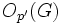# Equivalence of definitions of group of Glauberman type for a prime

This article gives a proof/explanation of the equivalence of multiple definitions for the term group of Glauberman type for a prime
View a complete list of pages giving proofs of equivalence of definitions

## Statement

Suppose$G$ is a finite group and$p$ is a prime number. In the discussion below,$Z(J(P))$ denotes the subgroup obtained by applying the ZJ-functor to$P$. The ZJ-functor is defined as the center of the Thompson subgroup$J(P)$, which in turn is defined as the join of abelian subgroups of maximum order. The following are equivalent:

1. For one (and hence every)$p$-Sylow subgroup$P$ of$G$,$G = O_{p'}(G)N_G(Z(J(P)))$: Here,$O_{p'}(G)$ denotes the$p'$-core of$G$,
2. For one (and hence every)$p$-Sylow subgroup$P$ of$G$, the image of$Z(J(P))$ in the quotient$G/O_{p'}(G)$ is a normal subgroup of$G/O_{p'}(G)$.
3. For one (and hence every)$p$-Sylow subgroup$Q$ of$K = G/O_{p'}(G)$,$Z(J(Q))$ is a normal subgroup of$K$.
4. For one (and hence every)$p$-Sylow subgroup$Q$ of$K = G/O_{p'}(G)$,$Z(J(Q))$ is a characteristic subgroup of$K$.

## Facts used

1. Equivalence of definitions of characteristic p-functor whose normalizer generates whole group with p'-core

## Proof

The proof follows directly from Fact (1), where the characteristic p-functor that we use is the ZJ-functor.